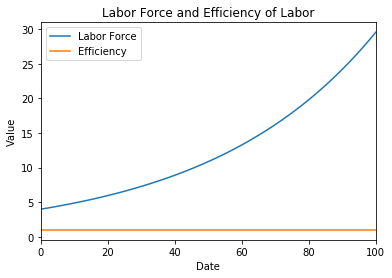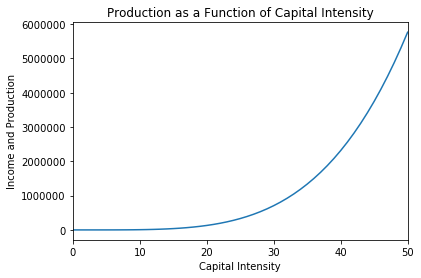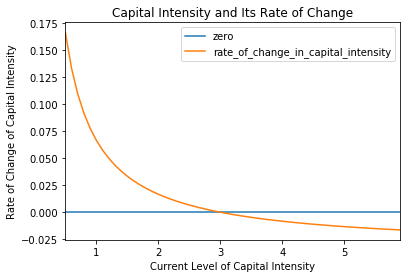## **2. The Basics of the Model** ¶

### **2.1. Preliminaries** ¶

#### **2.1.1. The Production Function** ¶

The first behavioral relationship in the Basic Solow Growth Model is the production function: the relationship between the economy's level of income and production $Y$ and its three determinants: the labor force $L$, the efficiency of labor $E$, and the economy's capital-intensity $\kappa$, which is measured by the quotient of the economy's capital stock $K$ and its level of income and production $Y$:

(2.1.1) $\kappa = \frac{K}{Y}$

**2.1.1.1. Satisfy Three Rules of Thumb**: We want this behavioral relationship to satisfy three rules of thumb:

1. A proportional increase in the economy's capital intensity $\kappa = K/Y$, measured by the capital stock divided by total production, will carry with it the same (smaller) proportional increase in income and production no matter how rich and productive the economy is. A 1% increase in capital intensity will always increase income and production by the same proportional amount.

2. If two economies have the same capital intensity, defined as the same capital-output ratio $\kappa$, and have the same level of technology- and organization-driven efficiency-of-labor $E$, then the ratio of their levels of income and output will be equal to the ratio of their labor forces $L$.

3. If two economies have the same capital intensity, defined as the same capital-output ratio $\kappa$, and have the same labor forces, then the ratio of their levels of income and output will be equal to the ratio of their technology- and organization-driven efficiencies-of-labor $E$.

There is one and only one way to write an algebraic expression that satisfies these two rule-of-thumb conditions. It is:

(2.1.2) $Y = \kappa^\theta E L$

or with a lower-case $y$ denoting income and production per worker:

(2.1.3) $y = \kappa^\theta E$

Note: You might object that this is circular: output and income $Y$ depend on capital intensity $\kappa$ defined as the capital-output ratio $K/Y$, but how can you calculate $\kappa$ and thus calculate $Y$ when you need to know $Y$ to calculate $\kappa$? If this worries you, you could start elsewhere, with a different-looking production function:

(2.1.4) $Y = K^\alpha (E L)^{1-\alpha}$

Then divide both sides by $Y^\alpha$:

(2.1.5) $\frac{Y}{Y^\alpha} = \frac{K^\alpha}{Y^\alpha} (E L)^{1-\alpha}$

(2.1.6) $Y^{1-\alpha} = \left(\frac{K}{Y}\right)^\alpha (E L)^{1-\alpha}$

(2.1.7) $Y^{1-\alpha} = \kappa^\alpha (E L)^{1-\alpha}$

(2.1.8) $Y^{\left(\frac{1-\alpha}{1-\alpha}\right)} = \kappa^{\left(\frac{\alpha}{1-\alpha}\right)} (E L)^{\left(\frac{1-\alpha}{1-\alpha}\right)}$

(2.1.9) $Y = \kappa^{\left(\frac{\alpha}{1-\alpha}\right)} E L$

which is exactly the same as (2.1.2), once we define $\alpha = \theta/(1+\theta)$ and thus $\theta = \alpha/(1-\alpha)$.

For historical reasons, every single other book uses $\alpha$. Since $\theta = \alpha/(1-\alpha)$, $\alpha = \theta/(1+\theta)$, and $1 - \alpha = \theta/(1+\theta)$, moving back and forth between the forms is not a problem. We will work with $\theta$ as it produces simpler formulas.

**2.1.1.2. Why These Rules of Thumb?**: Why did Robert Solow back in 1956 look for an algebraic formula for his production function that would satisfy these three rules-of-thumb? Economists like to simplify, ruthlessly, when they can get away with it. Rule-of-thumb (1) is a simplifying assumption: an intellectual bet that the process of aggregate economic growth is likely to look very similar as an economy goes from an income-per-capita level of 10,000 to 20,000 dollars per worker per year as when it goes from 40,000 to 80,000 dollars per worker per year. It is worth making only as long as that is in fact true—that the similarities in the aggregate overall economic growth process in different decades and at different income-per-worker levels outweigh the differences. If that were not or were to cease being the case, we should drop that rule-of-thumb assumption. So far, so good.

Rule-of-thumb (2) is simply that holding other things—capital intensity and efficiency-of-labor—constant, you can always duplicate what you are doing and produce and earn twice as much. Again, it is worth making only as long as that is in fact true, or approximately true. Again, so far, so good.

Rule-of-thumb (3) is a recognition that better-organized economies making better use of technology will be more productive: it is best thought of as a definition of how we are going to construct our quantitative index of the level of applied technological knowledge combined with efficient economic organization—the variable $E$ that we call the efficiency-of-labor. It has no deeper implications.

#### **2.1.2. Investment and Capital Accumulation** ¶

Following economists' custom of ruthless simplification, assume that individuals, households, and businesses desire to save a fraction $s$ of their income $Y$, so that total savings are:

(2.1.10) $S = sY$

We call s the economy’s saving rate or, more completely, its saving-investment rate (to remind us that s is measuring both the flow of saving into the economy’s financial markets and also the share of total production that is invested and used to build up and increase the economy’s capital stock).

Assume that there are no problems in translating individuals', households', and businesses' desires to save some of their income $Y$ into investment I:

(2.1.11) $I = S = sY$

While the saving-investment rate s is constant, the economy's capital stock K is not. It changes from year to year from investment and also from depreciation $\Delta$:

(2.1.12) $\frac{dK}{dt} = I - \Delta$

Assume that:

(2.1.13) $\Delta = \delta K$

Each year a fraction $\delta$ of the existing capital stock depreciates and wears out, so that the rate of change of the capital stock is:

The growth of the economy's capital stock $K$ is thus determined by investment, a share $s$ of income $Y$, minus depreciation, a share $\delta$ of the current capital stock $K$:

(2.1.14) $\frac{dK}{dt} = sY - \delta K = \left( \frac{s}{\kappa} - \delta \right)K$

We typically assume that s is constant. We do, however, think about the consequences of its taking a permanent upward or downward jump at some particular moment of time. The background assumption, however—made because it makes formulas much simpler—will always be that s will then remain at its jumped-to value as far as we look into the future.

#### **2.1.3. The Labor Force and the Efficiency-of-Labor** ¶

If the labor force L were constant and technological and organizational progress plus education that add to the efficiency-of-labor E were non-existent, we could immediately move on. But the economy’s labor force grows as more people turn 18 or so and join the labor force than retire, and as immigrants continue to arrive. And the efficiency of labor rises as science and technology progress, people keep thinking of new and more efficient forms of business organization, and people go to school and learn on the job

We assume—once again making a simplifying leap—that the economy’s labor force L's proportional rate $g_L$ is a constant n.

Note that n is not the same across countries. Note that it can and does shift over time in any one country. Since we want to tackle simple cases first, our background assumption will be that n is constant now as far as we can see into the future. But we will drop and vary this when we want to.

Thus between this year and the next the labor force grows according to the formula:

(2.1.15) $\frac{dL}{dt} = g_LL = nL$

Next year’s labor force will thus be n percent higher than this year’s labor force.

We also assume—once again making a simplifying leap—that the economy’s efficiency of labor E's proportional growth rate g is a constant every year. Note that g is not the same across countries. Note that it can and does shift over time in any one country. But we want to tackle simple cases first. A constant efficiency-of-labor growth rate g is simple. Thus our background assumption will be that g is constant as far as we can see into the future. Then between this year and the next the efficiency of labor grows according to the formula:

(2.1.16) $\frac{dE}{dt} = gE$

#### **2.1.4. Gaining Intuition for n, g, L, E, Y, $\kappa$, and $\theta$** </font>¶

Immediately below this paragraph are two Python code cells. The purpose of these two code cells is to make the above algebra real. What does it mean to say that the proportional growth rate of the labor force $L$ is $n = 0.02$—2% per year? It means that the labor force would double every thirty-five years. What does it mean to say that labor efficiency E's proportional growth rate is $g = 0.05$—5% per year? It means that labor efficiency doubles every twelve years. What are the implications of $\theta = 1$? It means that if you were to compare two economies with no differences save that the capital-intensity $\kappa$ of one were twice that of the other, output and output per worker in the first would also be twice as great as in the other. What are the implications of $\theta = 3$? It means that if you were to compare two economies with no differences save that the capital-intensity $\kappa$ of one were twice that of the other, output and output per worker in the first would also be nine times as great as in the other. Play with the code cells—it is the only way to make the algebra real:

In :
# CODE CELL: GAINING INTUITION FOR n & g & L AND E
#
# getting a sense of how the Solow growth model
# works...
#
# what are the implications of saying that the
# labor force L increases at a gross rate n?
# Or that the efficiency-of-labor E increases
# at a growth rate g? or that the elasticity
# of output Y with respect to the economy's
# capital-intensity κ is a parameter θ?
# these code cells are for you to experiment,
# and find out. they set out simple finger
# exercises for you to investigate.
#
# this code cell allows you to explore what
# various values for the parameters n and g
# (and also initial values L_0 and E_0) really
# mean.
#

# ----
# BEGIN BLOCK
#
# change the right-hand-sides of the equations
# in this block to what you wish. simply substitute
# your own choices of values for L_0, n, E_0,
# g, and the length T of the period you want
# to examine. then execute this code cell, and
# see what results:

T = 100

L_0 = 4
n = 0.02
E_0 = 1
g = 0.00

# END BLOCK
# ----

import numpy as np
import pandas as pd
import matplotlib.pyplot as plt

L_series = [L_0]
E_series = [E_0]
L = L_0
E = E_0

for i in range(T):
L = L*np.exp(n)
E = E *np.exp(g)
L_series = L_series + [L]
E_series = E_series + [E]

n_and_g_df = pd.DataFrame()
n_and_g_df['Labor Force'] = L_series
n_and_g_df['Efficiency'] = E_series

ax = n_and_g_df.plot()
ax.set_title("Labor Force and Efficiency of Labor")
ax.set_xlabel("Date")
ax.set_ylabel("Value")

plt.show()In :
# CODE CELL: GAINING INTUITION FOR Y & θ & κ
#
# this code cell allows you to explore what
# various values for the parameter θ mean for
# the relationship between Y and κ.
#
# choose your values for the efficiency-of-
# labor E, for the lafor force L; choose a
# maximum value for capital-intensity κ and
# a value for θ; or accept the values given
# in the block. then execute this code cell,
# and see what results:

# ----
# BEGIN BLOCK
#
# change the right-hand-sides of the equations
# in this block to what you wish. simply substitute
# your own choices of values for L, E, θ, and κ_{max}—
# the maximum capital-intensity you want to examine.
# then execute this code cell, and see what results:

L = 1
E = 1
κ_max = 50
θ = 4

# END BLOCK
# ----

import numpy as np
import pandas as pd
import matplotlib.pyplot as plt

κ_series = 
Y_series = 

for κ in range(κ_max):
Y = κ**θ * E * L
κ_series = κ_series + [κ]
Y_series = Y_series + [Y]

Y_and_κ_df = pd.DataFrame()
Y_and_κ_df['capital_intensity'] = κ_series
Y_and_κ_df['production'] = Y_series

Y_and_κ_df.set_index('capital_intensity')

ax = Y_and_κ_df.production.plot()
ax.set_title("Production as a Function of Capital Intensity")
ax.set_xlabel("Capital Intensity")
ax.set_ylabel("Income and Production")

plt.show()### **2.2. The Equilibrium Condition** ¶

#### **2.2.1. Balanced Growth** ¶

Multiply the economy's capital-intensity $\kappa$ by the economy's level of total income and production $Y$ and you get the economy's capital stock $K$:

(2.2.1) $K = \kappa Y$

the amount of produced means of production that the economy has inherited from its past.

Now take the natural log of (2.1.1) and then take the time derivative of the result:

(2.2.2) $\ln(Y) = \theta\ln(\kappa) + \ln(L) + \ln(E)$

(2.2.3) $\frac{1}{Y}\frac{dY}{dt} = g_Y = \theta \left( \frac{1}{\kappa}\frac{d\kappa}{dt} \right) + \frac{1}{L}\frac{dL}{dt} + \frac{1}{E}\frac{dE}{dt}$

We assumed that the second term on the right-hand-side of (2.2.3) is n and that the third term is g. If the capital-intensity $\kappa$ is constant, then the left-hand-side will be equal to n+g: that will then be the proportional growth rate $g_Y$ of income and production $Y$. If $Y$ is growing at rate $n+g$ and $\kappa$ is constant, then the economy's capital-stock $K$ will also be growing at $n+g$. Everything will then be in balanced growth. And if the economy is in balanced growth it will stay there. And if the economy is not in balanced growth, it will head for a configuration that is.

#### **2.2.2. When is Capital-Intensity $\kappa$ Constant?** ¶

We had assumed that a constant fraction $s$ of income $Y$ was saved and invested to add to the capital stock. We had assumed that a share $\delta$ of the capital stock rusts and erodes and disappears each year. Thus we had assumed that the capital-stock $K$ was changing at:

(2.2.4) $\frac{dK}{dt} = sY - \delta K$

And the capital stock was growing at a proportional growth rate:

(2.2.5) $\frac{1}{K}\frac{dK}{dt} = g_{K} = \frac{s}{\kappa} - \delta$

If we take the time derivative of (2.2.1) and substitute it into (2.2.3) we find:

(2.2.6) $\frac{1}{Y}\frac{dY}{dt} = g_Y = \theta \left( \frac{1}{K}\frac{dK}{dt} - \frac{1}{Y}\frac{dY}{dt} \right) + \frac{1}{L}\frac{dL}{dt} + \frac{1}{E}\frac{dE}{dt}$

(2.2.7) $(1+\theta)\frac{1}{Y}\frac{dY}{dt} = \theta \left( \frac{s}{\kappa} - \delta \right) + n + g$

So the proportional rate of growth of capital-intensity $\kappa$ is:

(2.2.8) $\frac{1}{\kappa}\frac{d\kappa}{dt} = g_\kappa = \frac{s}{\kappa} - \delta - \left( \frac{\theta}{1+\theta} \right)\left( \frac{s}{\kappa} - \delta \right) - \frac{n + g}{1+\theta}$

(2.2.9) $\frac{1}{\kappa}\frac{d\kappa}{dt} = \frac{s/\kappa - (n+g+\delta)}{1+\theta}$

Thus the capital-stock will be growing at the rate $n+g$ required for balanced growth if and only if:

(2.2.10) $\frac{s}{\kappa} - \delta = n + g$

#### **2.2.3. Gaining Intuition with Respect to the Rate of Change of Capital-Intensity $\kappa$** ¶

Immediately below this paragraph is a Python code cell to help you gain some intuition with respect to what equation (2.2.9) is telling us about the rate of change of the capital-intensity $\kappa$ of an economy following the Solow growth model. Once again, play with the code cell—it is the only way to make the algebra real:

In :
# CODE CELL: 𝜅, 𝜅*, AND THE DYNAMIC BEHAVIOR OF CAPITAL INTENSITY
#
# this code cell plots how the proportional growth rate
# g_𝜅 of capital-intensity 𝜅 varies with the level
# of capital-intensity 𝜅.
#
# it will show you that the growth rate of capital-
# intensity 𝜅 is equal to zero if and only if:
#
#    𝜅 = 𝜅^* = s/(n+g+𝛿)
#
# either accept the values given below for s, n, g, and
# 𝛿, or substitute your own in the relevant code lines
# in the block. then execute this code cell, and see
# what results:

# ----
# BEGIN BLOCK
#
# change the right-hand-sides of the equations
# in this block to what you wish. simply substitute
# your own choices of values for s, n, g, 𝛿, and θ.
# then execute this code cell, and see what results:

s = 0.30
n = 0.01
g = 0.015
𝛿 = 0.075
θ = 2

# END BLOCK
# ----

import numpy as np
import pandas as pd
import matplotlib.pyplot as plt

𝜅_star = s/(n+g+𝛿)
𝜅_max = 2*𝜅_star
𝜅_min = 0.5

d𝜅_series = []
𝜅_series = []
zero_series = []

for j in range(round(10*𝜅_min), 10*round(𝜅_max)):
𝜅 = j/10
d𝜅_series = d𝜅_series + [(s/𝜅 - (n+g+𝛿))/(1+θ)]
𝜅_series = 𝜅_series + [𝜅]
zero_series = zero_series + 

𝜅_and_d𝜅_df = pd.DataFrame()
𝜅_and_d𝜅_df['rate_of_change_in_capital_intensity'] = d𝜅_series
𝜅_and_d𝜅_df['capital_intensity'] = 𝜅_series
𝜅_and_d𝜅_df['zero'] = zero_series

ax = plt.gca()

𝜅_and_d𝜅_df.plot(kind='line', x='capital_intensity', y='zero', ax=ax)
𝜅_and_d𝜅_df.plot(kind='line', x='capital_intensity',
y='rate_of_change_in_capital_intensity',
title = 'Capital Intensity and Its Rate of Change',
ax=ax)

ax.set_xlabel("Current Level of Capital Intensity")
ax.set_ylabel("Rate of Change of Capital Intensity")

plt.show()## **Lecture Notes: The Solow Growth Model** ¶